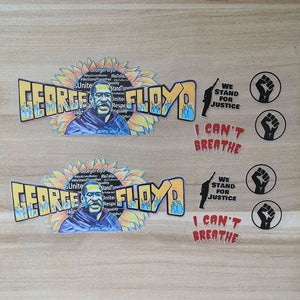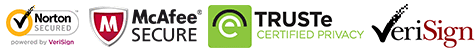FedEx will stop during Oct 1-8
FedEx will stop during Oct 1-8# Black Lives Matter Iron on Patches for Custom Air Force 1 George Floyd

Regular price \$15.00 \$9.90 Save \$5.10

ONLY 199938 LEFT

 Hours   Minutes   Seconds

Sale Ends Once The Timer Hits Zero!

ONLY 199938 LEFT

Get these George Floyd iron on patches to DIY your own BLM shoes. Best size for DIY or custom Air Force 1 & Vans.

<<<<<<<<<<<<<<<<<<<<<<<<<<<<<<<<<<<<<<<<<<<<<<<<<<<<<<<<

The set includes

2 Floyd

2 we stand for justice

2 i can't breathe

2 fight together

The shoes are not included in the set.

<<<<<<<<<<<<<<<<<<<<<<<<<<<<<<<<<<<<<<<<<<<<<<<<<<<<<<<

Size:

George Floyd:

width 11.2cm

Height: 5 cm

other small signs has not measure, please check the sample shoes

<<<<<<<<<<<<<<<<<<<<<<<<<<<<<<<<<<<<<<<<<<<<<<<<<<<<<<<

### How to use:

1. Position the George Floyd and other small patches on the shoes with tape

2. Set your iron temperature to 135-150℃, and iron directly on the smooth side

3. If use normal iron, please turn off the steam, keep the temperature above, use the tip and be careful and patient, for it's heavy to control, better use the mini iron.

<<<<<<<<<<<<<<<<<<<<<<<<<<<<<<<<<<<<<<<<<<<<<<<<<<<<<<<

### Free shipping

people are currently looking at this product

Estimated delivery time 7-15 days

Guaranteed Safe Checkout